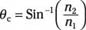##### Optics For Dummies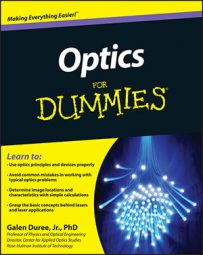Reflection and refraction are two processes that change the direction light travels. Using the equations for calculating reflection and refraction, you can predict where rays encountering a surface will go — whether they reflect or refract (bounce off the surface or bend through it) — which is an important concept in the study of optics. The following equations help you determine reflection and refraction angles:

• The law of reflection: The law of reflection shows the relationship between the incident angle and the reflected angle for a ray of light incident on a surface. The angles are measured relative to the surface normal (a line that is perpendicular to the surface), not relative to the surface itself. Here's the formula: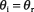• The index of refraction: This quantity describes the effect of atoms and molecules on the light as it travels through a transparent material. Use this basic formula for the index of refraction: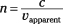• Snell's law or the law of refraction: Snell's law shows the relationship between the incident angle and the transmitted angle (refracted angle) for a ray of light incident on a surface of a transparent material. You can see how Snell's law works in the following formula: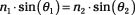• The critical angle for total internal reflection: Total internal reflection is the situation where light hits and reflects off the surface of a transparent material without transmitting through the surface. It utilizes the critical angle (the minimum angle of incidence where total internal reflection takes place.). For total internal reflection to occur, the light must start in the material with the higher index. Here's the formula: